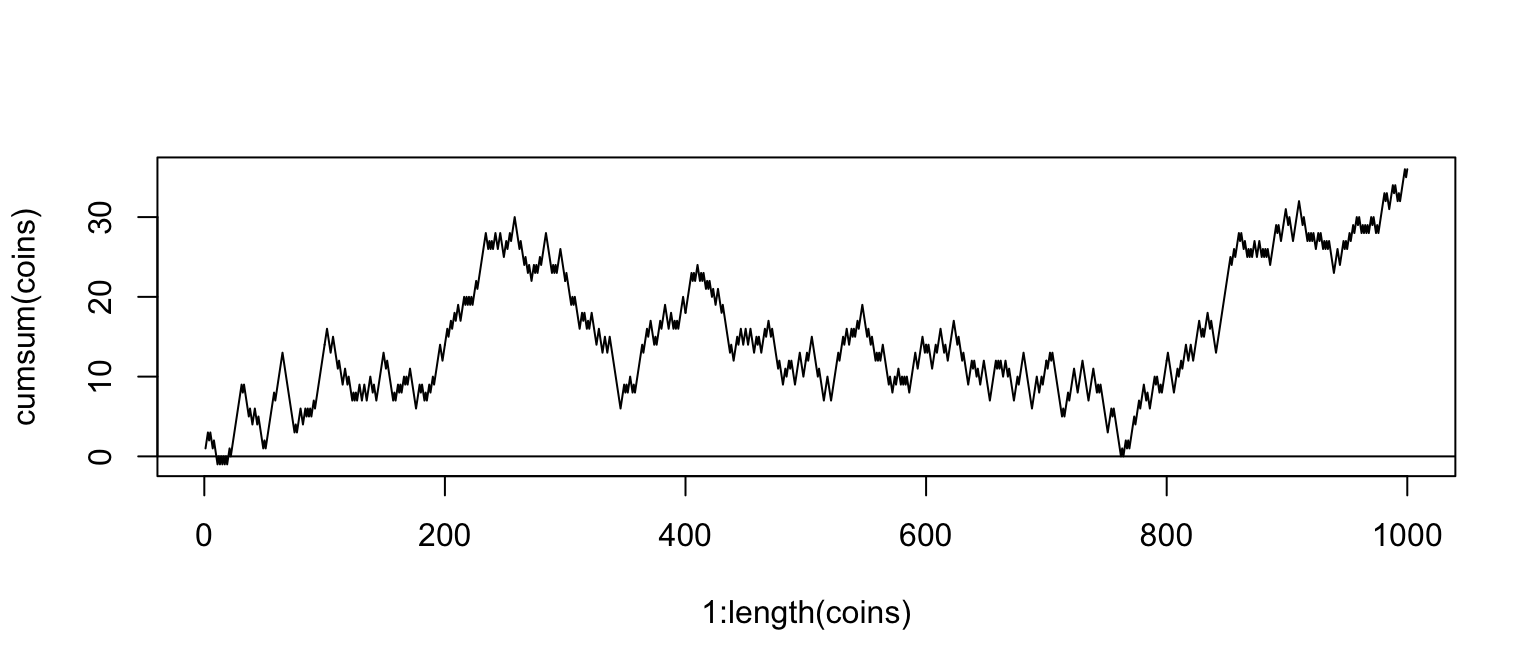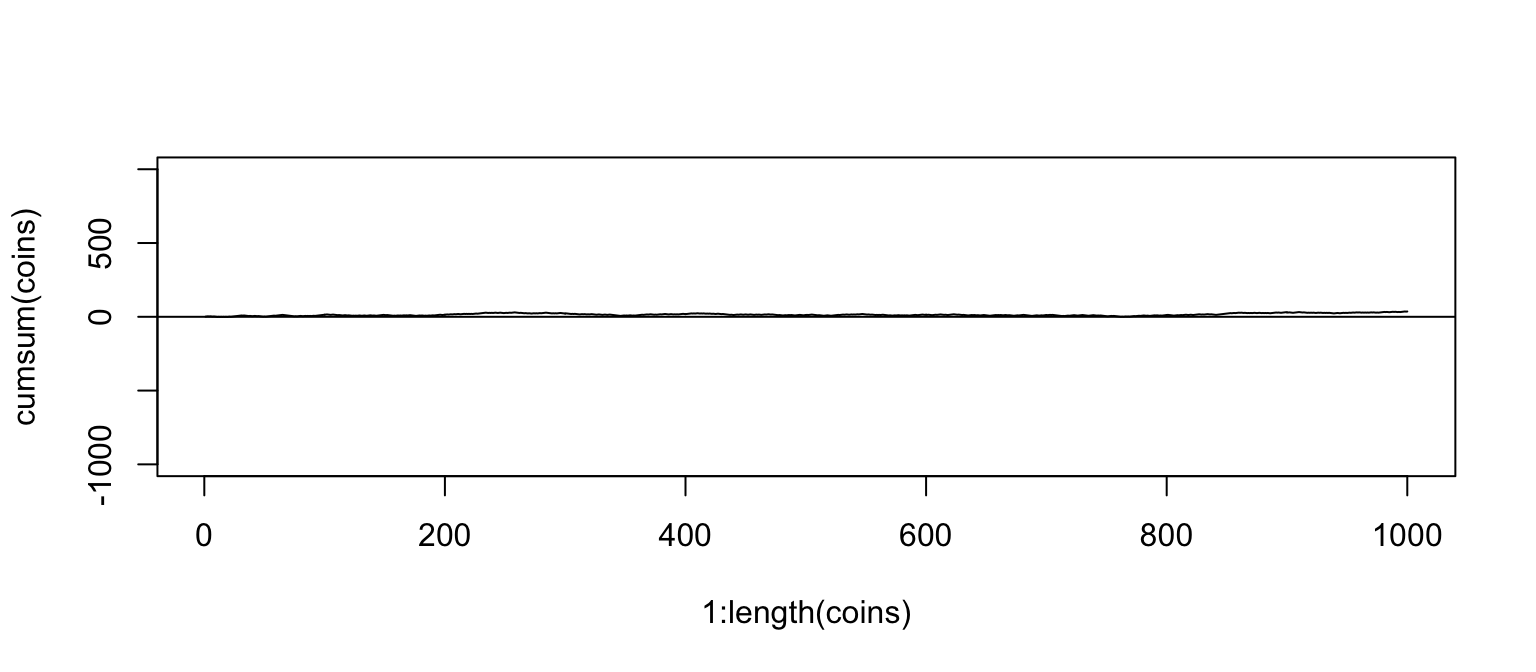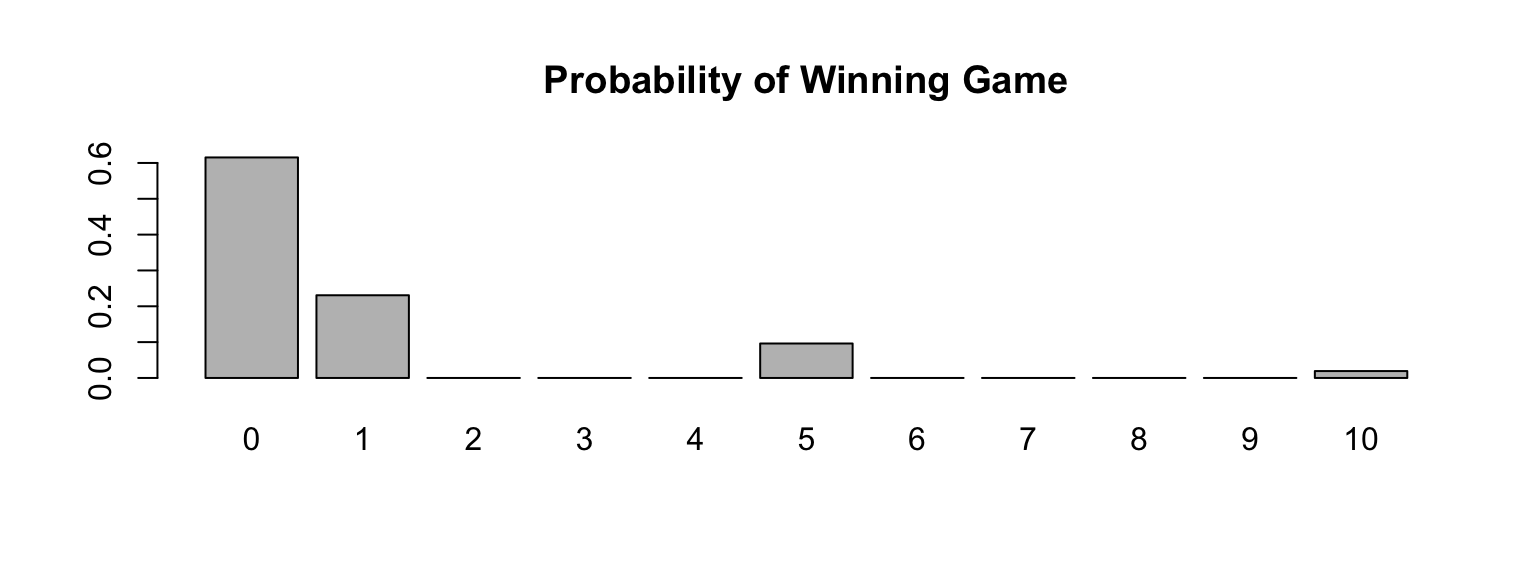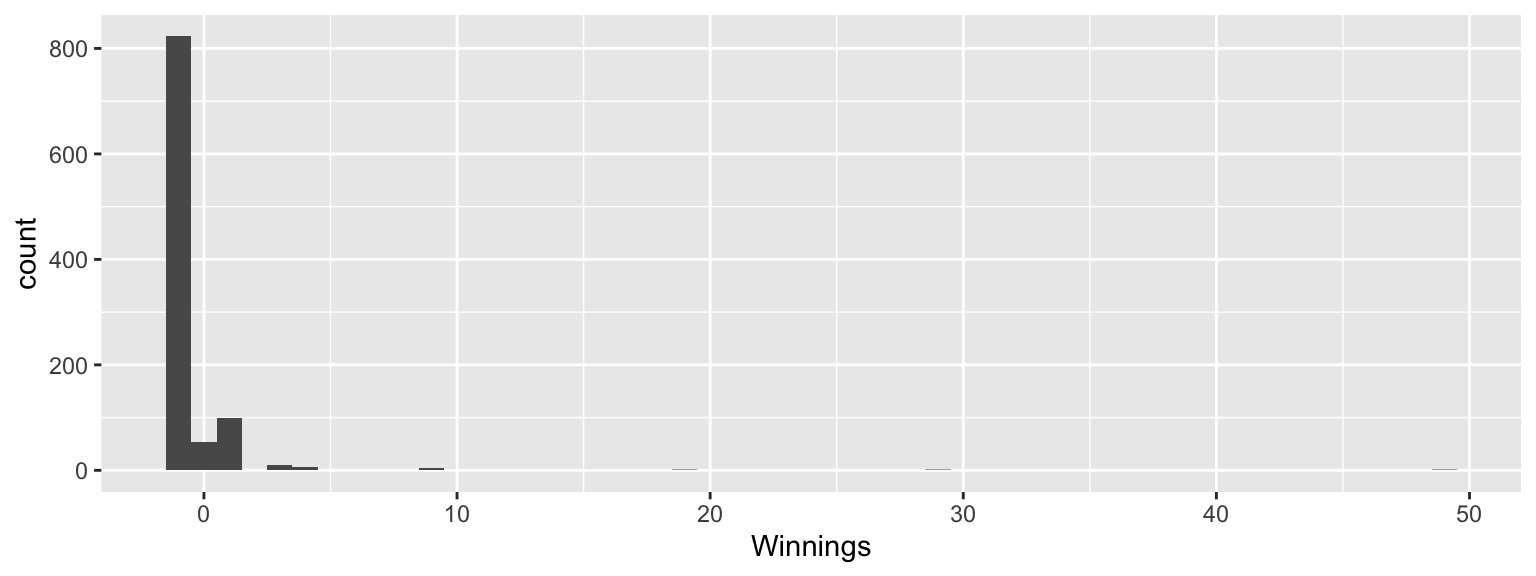February 12, 2020

## Announcements

• NY R Conference will be May 8th and 9th. More info here: https://rstats.ai/nyr/ (You can use code landeR2020 for a 20% discount).
• Two new posts on course website:

## Probability

There are two key properties of probability models:

1. P(A) = The probability of event A
2. $$0 \le P(A) \le 1$$

This semester we will examine two interpretations of probabilty:

• Frequentist interpretation: The probability of an outcome is the proportion of times the outcome would occur if we observed the random process an infinite number of times.

• Bayesian interpretation: A Bayesian interprets probability as a subjective degree of belief: For the same event, two separate people could have different viewpoints and so assign different probabilities. Largely popularized by revolutionary advance in computational technology and methods during the last twenty years.

## Law of Large Numbers

Law of large numbers states that as more observations are collected, the proportion of occurrences with a particular outcome, $${\hat{p}}_n$$, converges to the probability of that outcome, $$p$$.

## Law of Large Numbers (cont)

￼When tossing a fair coin, if heads comes up on each of the first 10 tosses, what do you think the chance is that another head will come up on the next coin toss? 0.5, less 0.5, or greater 0.5?

## Law of Large Numbers (cont)

￼When tossing a fair coin, if heads comes up on each of the first 10 tosses, what do you think the chance is that another head will come up on the next coin toss? 0.5, less 0.5, or greater 0.5?

• The probability is still 0.5, or there is still a 50% chance that another head will come up on the next toss.
• The coin is not “due”" for a tail.
• The common misunderstanding of the LLN is that random processes are supposed to compensate for whatever happened in the past; this is just not true and is also called gambler’s fallacy (or law of averages).

## Coin Toss Demo

library(DATA606)
shiny_demo('gambler')

## Coin Tosses

coins <- sample(c(-1,1), 1000, replace=TRUE)
plot(1:length(coins), cumsum(coins), type='l')
abline(h=0)## Coin Tosses (Full Range)

plot(1:length(coins), cumsum(coins), type='l', ylim=c(-1000, 1000))
abline(h=0)## Disjoint and non-disjoint outcomes

Disjoint (mutually exclusive) outcomes: Cannot happen at the same time.

• The outcome of a single coin toss cannot be a head and a tail. A student both cannot fail and pass a class.
• A single card drawn from a deck cannot be an ace and a queen.

Non-disjoint outcomes: Can happen at the same time.

• A student can get an A in Stats and A in Econ in the same semester.

## Probability Distributions

A probability distribution lists all possible events and the probabilities with which they occur.

• The probability distribution for the gender of one kid:
Event Male Female
Probabilty 0.5 0.5

Rules for probability distributions:

1. The events listed must be disjoint
2. Each probability must be between 0 and 1
3. The probabilities must total 1

## Probabilty Distrubtions (cont.)

The probability distribution for the genders of two kids:

Event MM FF MF FM
Probability 0.25 0.25 0.25 0.25

## Independence

Two processes are independent if knowing the outcome of one provides no useful information about the outcome of the other.

• Knowing that the coin landed on a head on the first toss does not provide any useful information for determining what the coin will land on in the second toss. → Outcomes of two tosses of a coin are independent.
• Knowing that the first card drawn from a deck is an ace does provide useful information for determining the probability of drawing an ace in the second draw. → Outcomes of two draws from a deck of cards (without replacement) are dependent.

## Checking for Independence

If P(A occurs, given that B is true) = P(A | B) = P(A), then A and B are independent.

• P(protects citizens) = 0.58
• P(randomly selected NC resident says gun ownership protects citizens, given that the resident is white) = P(protects citizens | White) = 0.67
• P(protects citizens | Black) = 0.28
• P(protects citizens | Hispanic) = 0.64

P(protects citizens) varies by race/ethnicity, therefore opinion on gun ownership and race ethnicity are most likely dependent.

## Lottery

shiny_demo('lottery')

## Random Variables

A random variable is a numeric quantity whose value depends on the outcome of a random event

• We use a capital letter, like X, to denote a random variable
• The values of a random variable are denoted with a lowercase letter, in this case x
• For example, P(X = x)

There are two types of random variables:

• Discrete random variables often take only integer values
Example: Number of credit hours, Difference in number of credit hours this term vs last
• Continuous random variables take real (decimal) values
Example: Cost of books this term, Difference in cost of books this term vs last

## Expectation

• We are often interested in the average outcome of a random variable.
• We call this the expected value (mean), and it is a weighted average of the possible outcomes

$\mu =E\left( X \right) =\sum _{ i=1 }^{ k }{ { x }_{ i }P\left( X={ x }_{ i } \right) }$

￼In a game of cards you win $1 if you draw a heart,$5 if you draw an ace (including the ace of hearts), $10 if you draw the king of spades and nothing for any other card you draw. Write the probability model for your winnings, and calculate your expected winning. Event X P(X) X P(X) Heart (not Ace) 1 12/52 12/52 Ace 5 4/52 20/52 King of Spades 10 1/52 10/52 All else 0 35/52 0 Total $$E(X) = \frac{42}{52} \approx 0.81$$ ## Expected value of a discrete random variable cards <- data.frame(Event = c('Heart (not ace)','Ace','King of Spades','All else'), X = c(1, 5, 10, 0), pX = c(12/52, 5/52, 1/52, 32/52) ) cards$XpX <- cards$X * cards$pX
cards2 <- rep(0, 11)
cards2[cards$X + 1] <- cards$pX
names(cards2) <- 0:10
barplot(cards2, main='Probability of Winning Game')## Estimating Expected Values with Simulations

tickets <- as.data.frame(rbind(
c(    '$1', 1, 15), c( '$2',    2,     11),
c(    '$4', 4, 62), c( '$5',    5,    100),
c(   '$10', 10, 143), c( '$20',   20,    250),
c(   '$30', 30, 562), c( '$50',   50,   3482),
c(  '$100', 100, 6681), c( '$500',  500,  49440),
c('$1500', 1500, 375214), c('$2500',  2500, 618000)
), stringsAsFactors=FALSE)
names(tickets) <- c('Winnings', 'Value', 'Odds')
tickets$Value <- as.integer(tickets$Value)
tickets$Odds <- as.integer(tickets$Odds)

odds <- sample(max(tickets$Odds), 1000, replace=TRUE) vals <- rep(-1, length(odds)) for(i in 1:nrow(tickets)) { vals[odds %% tickets[i,'Odds'] == 0] <- tickets[i,'Value'] - 1 } head(vals, n=20) ##  -1 -1 -1 1 0 -1 -1 -1 -1 -1 -1 -1 -1 -1 0 -1 3 -1 -1 -1 mean(vals) ##  -0.533 ## Estimating Expected Values with Simulations ggplot(data.frame(Winnings=vals), aes(x=Winnings)) + geom_bar(binwidth=1) ## Warning: geom_bar() no longer has a binwidth parameter. Please use ## geom_histogram() instead.## Expected Value of Lottery Example $\mu =E\left( X \right) =\sum _{ i=1 }^{ k }{ { x }_{ i }P\left( X={ x }_{ i } \right) }$ tickets ## Winnings Value Odds xPx ## 1$1     1     15 0.066666667
## 2        $2 2 11 0.181818182 ## 3$4     4     62 0.064516129
## 4        $5 5 100 0.050000000 ## 5$10    10    143 0.069930070
## 6       $20 20 250 0.080000000 ## 7$30    30    562 0.053380783
## 8       $50 50 3482 0.014359563 ## 9$100   100   6681 0.014967819
## 10     $500 500 49440 0.010113269 ## 11$1500  1500 375214 0.003997719
## 12    $2500 2500 618000 0.004045307 sum(tickets$xPx) - 1 # Expected value for one ticket
##  -0.3862045

## Expected Value of Lottery Example (cont)

sum(tickets$xPx) - 1 # Expected value for one ticket ##  -0.3862045 Simulated nGames <- 1 runs <- numeric(10000) for(j in seq_along(runs)) { odds <- sample(max(tickets$Odds), nGames, replace = TRUE)
vals <- rep(-1, length(odds))
for(i in 1:nrow(tickets)) {
vals[odds %% tickets[i,'Odds'] == 0] <- tickets[i,'Value'] - 1
}
runs[j] <- cumsum(vals)[nGames]
}
mean(runs)
##  -0.4385